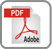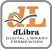# COMPUTATIONAL RESULTS FOR INTEGRAL EQUATIONS IN SPACE-TIME

Hącia Lechosław

Institute of Mathematics, Poznań University of Technology
Piotrowo 3A, 60-965 Poznań, Poland

### Abstract:

An important class of integral equations in space-time is discussed. The studied equations, called Volterra-Fredholm integral equations or integral equations of the mixed type are used in technology, mechanics, biology and the heat conduction theory. First, the Galerkin method is applied to the Fredholm integral equations in space-time. Next, it is used for the Volterra-Fredholm integral equations. The presented algorithm is illustrated by graphs and tables.

### References:

[ 1 ] H. Brunner, SIAM J. Numer. Anal. 27, 987 (1990).
 L. Hącia, Zeit Ang. Math. Mech. 415 (1996).
 L. Hącia, Demon. Math. 32. 795 (1999).
 J. P. Kauthen, Numer. Math. 56. 409 (1989).
 W. I. Tivončuk, Diff. Uravn. 2,9 1228 (1966) (in Russian).
 I. N. Tukalevska, II Sc. Conf. of Young Ukr. Math., Naukova Dumka, Kiev, 609 (1966)
(in Ukrainian).